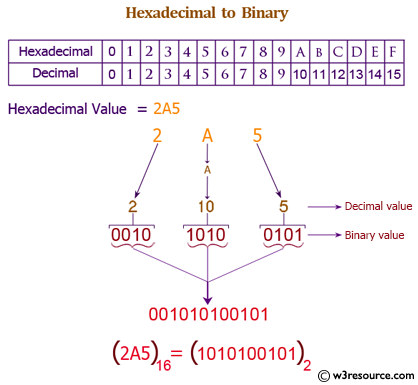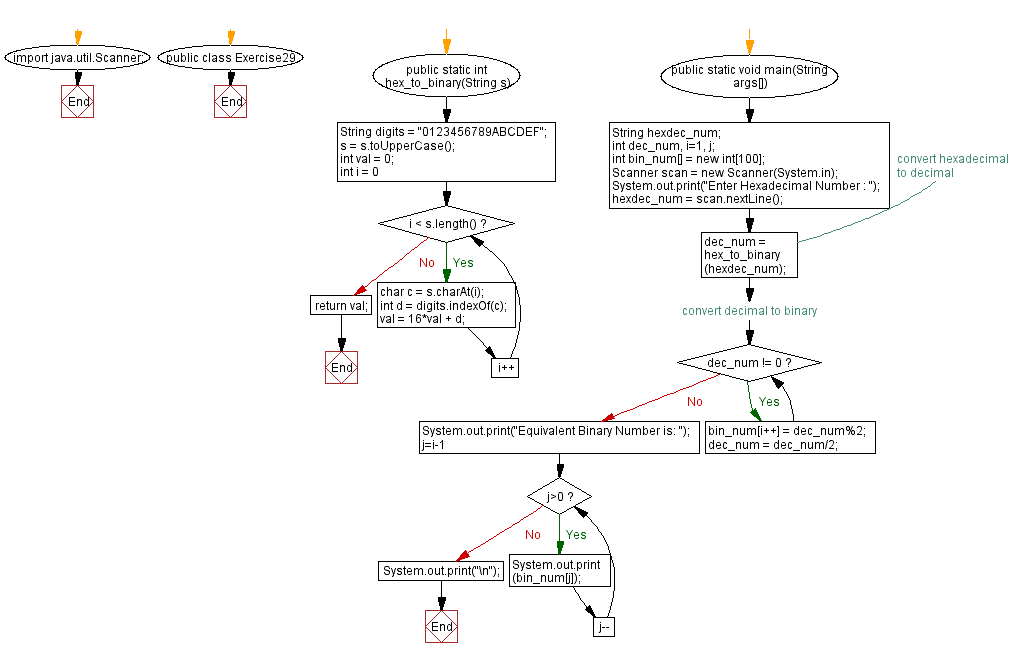﻿ Java exercises: Convert a hexadecimal to a binary number - w3resource# Java Exercises: Convert a hexadecimal to a binary number

## Java Basic: Exercise-29 with Solution

Write a Java program to convert a hexadecimal to a binary number.

Hexadecimal number: This is a positional numeral system with a radix, or base, of 16. Hexadecimal uses sixteen distinct symbols, most often the symbols 0-9 to represent values zero to nine, and A, B, C, D, E, F (or alternatively a, b, c, d, e, f) to represent values ten to fifteen.

Binary number: A binary number is a number expressed in the base-2 numeral system or binary numeral system. This system uses only two symbols: typically 1 (one) and 0 (zero).

Test Data:

Pictorial Presentation: Hexadecimal to Binary numberSample Solution:

Java Code:

``````import java.util.Scanner;
public class Exercise29 {
public static int hex_to_decimal(String s)
{
String digits = "0123456789ABCDEF";
s = s.toUpperCase();
int val = 0;
for (int i = 0; i < s.length(); i++)
{
char c = s.charAt(i);
int d = digits.indexOf(c);
val = 16*val + d;
}
return val;
}
public static void main(String args[])
{
String hexdec_num;
int dec_num, i=1, j;
int bin_num[] = new int;
Scanner scan = new Scanner(System.in);

hexdec_num = scan.nextLine();

/* convert hexadecimal to decimal */
dec_num = hex_to_decimal(hexdec_num);

/* convert decimal to binary */
while(dec_num != 0)
{
bin_num[i++] = dec_num%2;
dec_num = dec_num/2;
}

System.out.print("Equivalent Binary Number is: ");
for(j=i-1; j>0; j--)
{
System.out.print(bin_num[j]);
}
System.out.print("\n");
}
}
```
```

Sample Output:

```Enter Hexadecimal Number : 37
Equivalent Binary Number is: 110111
```

Flowchart:Java Code Editor:

What is the difficulty level of this exercise?

Test your Programming skills with w3resource's quiz.

﻿

## Java: Tips of the Day

countOccurrences

Counts the occurrences of a value in an array.

Use Arrays.stream().filter().count() to count total number of values that equals the specified value.

```public static long countOccurrences(int[] numbers, int value) {
return Arrays.stream(numbers)
.filter(number -> number == value)
.count();
}
```

Ref: https://bit.ly/3kCAgLb